数据结构--时间复杂度的算法

前前言

what  is  O?:"O"是数学符号，它的严格定义是"若T(n)和f(n)是定义在正整数集合上的两个函数，则T(n)=O(f(n))表示存在正的常数C和n0 ,使得当n≥n0时都满足0≤T(n)≤C?f(n)。"用容易理解的话说就是这两个函数当整型自变量n趋向于无穷大时，两者的比值是一个不等于0的常数。

3.大O表示法

1.用常数1来取代运行时间中所有加法常数。
2.修改后的运行次数函数中，只保留最高阶项
3.如果最高阶项存在且不是1，则去除与这个项相乘的常数。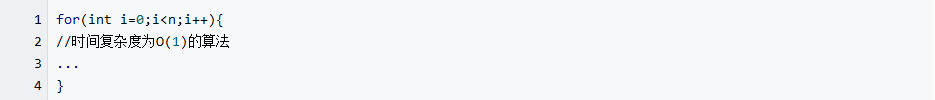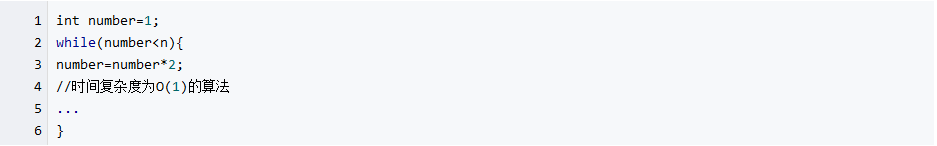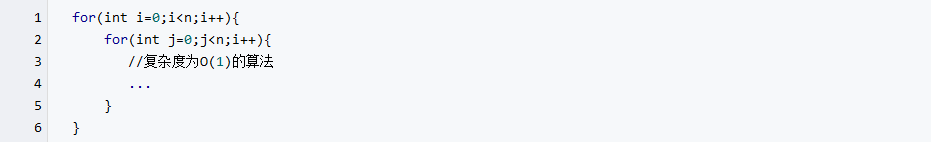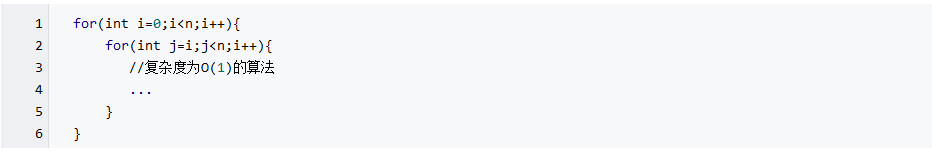n+(n-1)+(n-2)+(n-3)+……+1
=(n+1)+[(n-1)+2]+[(n-2)+3]+[(n-3)+4]+……
=(n+1)+(n+1)+(n+1)+(n+1)+……
=(n+1)n/2
=n(n+1)/2
=n²/2+n/2

f(n)=nlogn时，时间复杂度为O(nlogn)，可以称为nlogn阶。
f(n)=n³时，时间复杂度为O(n³)，可以称为立方阶。
f(n)=2ⁿ时，时间复杂度为O(2ⁿ)，可以称为指数阶。
f(n)=n!时，时间复杂度为O(n!)，可以称为阶乘阶。
f(n)=(√n时，时间复杂度为O(√n)，可以称为平方根阶。

4.复杂度的比较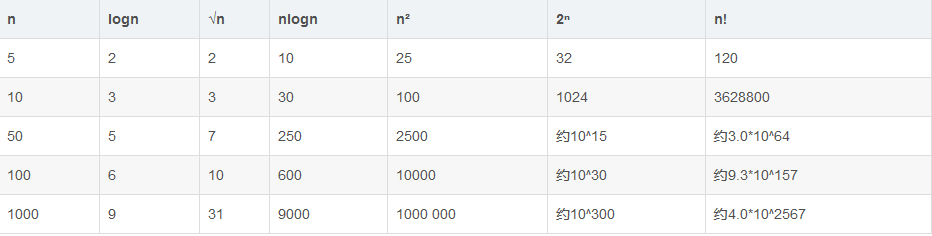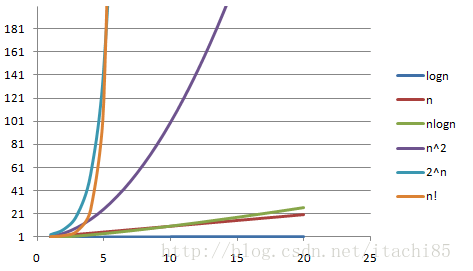posted on 2018-03-12 23:10  cavsc  阅读(3070)  评论(0编辑  收藏  举报